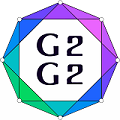#Graphs and Groups, Geometries and GAP (G2G2) Summer School - External Satellite Conference of 8ECM

27 June 2021 to 3 July 2021
Rogla, Slovenia
UTC timezone

## The Orbital Diameter of Primitive Permutation Groups

Not scheduled
20m
Rogla, Slovenia

#### Rogla, Slovenia

Hotel Planja, Rogla 1, 3214 Zreče, Slovenia
Oral presentation

### Speaker

Ms Kamilla Rekvenyi (Imperial College London)

### Description

Let $G$ be a group acting transitively on a finite set $\Omega$. Then $G$ acts on $\Omega\times \Omega$ componentwise. Define the orbitals to be the orbits of $G$ on $\Omega\times \Omega$. The diagonal orbital is the orbital of the form $\Delta=\{(\alpha,\alpha)\vert \alpha \in \Omega\}.$ The others are called non-diagonal orbitals. Let $\Gamma$ be a non-diagonal orbital. Define an orbital graph to be the non-directed graph with vertex set $\Omega$ and edge set $(\alpha,\beta)\in \Gamma$ with $\alpha,\beta\in \Omega$. If the action of $G$ on $\Omega$ is primitive, then all non-diagonal orbital graphs are connected. The orbital diameter of a primitive permutation group is the supremum of the diameters of its non-diagonal orbital graphs.

There has been a lot of interest in finding bounds on the orbital diameter of primitive permutation groups. In my talk I will outline some important background information and the progress made towards finding specific bounds on the orbital diameter. In particular, I will discuss some results on the orbital diameter of the groups of simple diagonal type and their connection to the covering number of finite simple groups. I will also discuss some results for affine groups, which provides a nice connection to the representation theory of quasisimple groups.

### Primary author

Ms Kamilla Rekvenyi (Imperial College London)